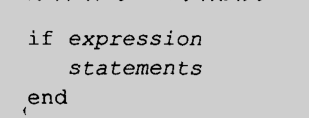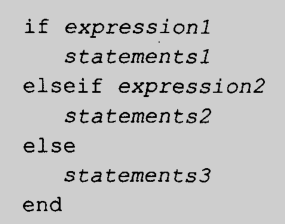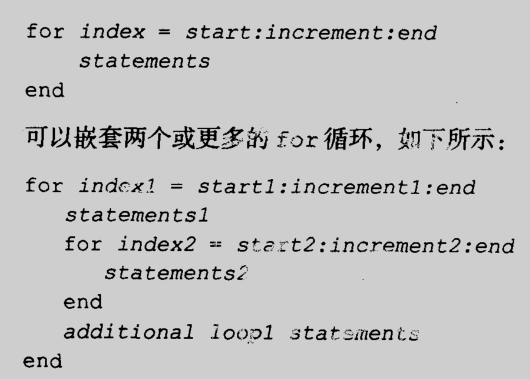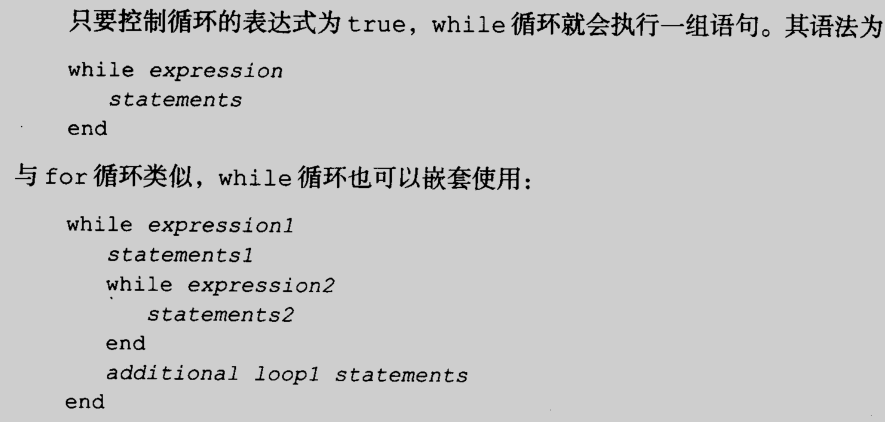• Matlab for循环 二维数组赋值时间过长问题
2021-08-08 00:12:54

for i=1:250

for j=1:250

a(i,j)=i*j;

end

end

这几行代码，跑起来需要将近30分钟

开始时候速度很快，但随着数组a的长度增加，速度越来越慢

有人说需要提前预置空间，如下

a=zeros(250)

for i=1:250

for j=1:250

a(i,j)=i*j;

end

end

但速度并没有大大提高

这时，我们可以采用一元数组求解，最后将所有解合起来,如下

a=zeros(250)

for i=1:250

for j=1:250

w(j)=i*j;

end

a(i,:)=w

end

这种方法可以大幅度减少时间消耗，大约3分钟左右即可跑完。算法
更多相关内容
• clear;clc;x=[2 3 4 5 7 8 10 11 14 15 16 18 19];y=[106。42 108。26 109。58 109。5 110 109。93 110。49 110。59 110。...%可以放在循环外%v=zeros(3,6);没有必要，需要的话可以，v=[];for n=1:...

clear;clc;

x=[2 3 4 5 7 8 10 11 14 15 16 18 19];

y=[106。42 108。26 109。58 109。5 110 109。93 110。49 110。

59 110。6 110。9 110。76 111 111。2];

x3=min(x):0。1:max(x);%可以放在循环外

%v=zeros(3,6);没有必要，需要的话可以，v=[];

for n=1:3

v(n,1:n 3)=polyfit(x,y,n 2);

y3(:,n)=polyval(v(n,1:n 3),x3);

text=strcat(num2str(n 2),'次多项式模拟函数为');

disp(text);

disp(poly2str(v(n,1:n 3),'x'));

end

%用多项式拟合一般效果都不好，用分式拟合效果好

fun=@(a,t) (a(1)*t。

^2 a(2)*t a(3))。/ (t a(4))

a0=[0。1 100 -100 -1];

a=nlinfit(x,y,fun,a0)

yf=fun(a,x3);

plot(x,y,'o',x3,y3,x3,yf,'*')

结果：

3次多项式模拟函数为

0。

0032566 x^3 - 0。12242 x^2 1。5113 x 104。4824

4次多项式模拟函数为

-0。00048007 x^4 0。023554 x^3 - 0。40963 x^2 3。

0473 x 102。0444

5次多项式模拟函数为

9。6416e-005 x^5 - 0。0054588 x^4 0。11758 x^3 - 1。2012 x^2 5。9223 x

98。

5719

fun =

@(a,t)(a(1)*t。^2 a(2)*t a(3))。/(t a(4))

a =

0。0541 110。

2197 -117。8171 -1。0335。

全部

展开全文• 问题是如何对 由classdef 产生的对象 构建的数组赋值的问题。最近用matlab做了一些面向对象的开发工作，感觉很爽，但是遇到一个对于类数组的赋值问题，一直无解，不知道是不是matlab不支持。我用的是 matlab r2008a ...

不好意思 ， 上次自己发的那个帖子找不到了。重新发一个。

问题是如何对 由classdef 产生的对象 构建的数组赋值的问题。

最近用matlab做了一些面向对象的开发工作，感觉很爽，但是遇到

一个对于类数组的赋值问题，一直无解，不知道是不是matlab不支持。

我用的是 matlab r2008a (7.06.0.324)

问题如下：element 是一个类，有一些基本属性；

container是另外一个类，container其中的一个属性 “con_ele” ，是element的数组；

有没有办法不用for 可以一次性对container中所有的"con_ele" 的某一个属性，例如 con_ele.ns

进行赋？

目前可以一次性都取出来，无论是做成数组还是cell，但是不能一次性赋值，只能用

for循环，总是会报“??? Insufficient number of outputs from function on right hand side of equal sign

测试用例如下：

element.m     element     类定义文件

container.m   container  类定义文件

testbench.m       测试平台文件

附加的小问题：这里如果用for会很影响效率吗？

谢谢各位大侠！

试验代码：

--------element.m --------------

classdef element

properties

ns,

nt,

nv,

nu,

end

methods

function obj=element()

obj.ns =0;

obj.nt =1;

obj.nv =2;

obj.nu =3;

end

end

end

-----------end element.m-------------

------------container.m---------------

classdef container

properties

cona,

conb,

con_ele

end

methods

function obj = container(s)

obj.cona = 3.14;

obj.conb = 6.28;

obj.con_ele = repmat(element,s,1);

end

end

end

------------end container.m----------

----------testbench.m------------

%test_classarray

clear;clc;

size = 10;

test_containter = container(size);

%different ways to get container.con_ele.nt value

ele_nt_mat = [test_containter.con_ele.nt];

ele_nt_cell = {test_containter.con_ele.nt};

%Question:

%How to change all the value of test_containter.con_ele.nt

%Change one value, THIS WORKS.

test_containter.con_ele(1).nt = 9;

%Methods to change more than one value I have tried.

%  They *WON'T* work

test_containter.con_ele(1:3).nt = [9,9,9];

test_containter.con_ele(1:3).nt = [9,9,9]';

test_containter.con_ele(1:3).nt = {9,9,9};

test_containter.con_ele(1:3).nt = {9,9,9}';

%QUESTION

%  Is there some ways to solve this problem without for loop?

---------------end testbench.m---------------

展开全文• 想问一下怎么在循环里让一个数组里的数挨个赋值给x 比如有一串数组是日价格 算当日价格减去某定值
• 向量索引 1，（向量）数组创建与元素存取 >> v = [1,3,5,7,8] v = 1 3 5 7 8 >> a = v(1),v(3) = 9 a = 1 v = 1 3 9 7 8 2，转置运算 >...

### 向量索引

1，（向量）数组创建与元素存取

  >> v = [1,3,5,7,8]
v =
1     3     5     7     8
>> a = v(1),v(3) = 9
a =
1
v =
1     3     9     7     8


2，转置运算

>> v = [1,3,5,7,8];
>> w =  v.'
w =
1
3
5
7
8


3，多个数据进行存取
其中end表示向量中的最后一个元素

>> v = [1,3,5,7,8];
>> v(1:3)
ans =
1     3     5
>> v(2:end)
ans =
3     5     7     8


4，行向量转列向量

>> v = [1,3,5,7,8];
>> v(:)
ans =
1
3
5
7
8


5，索引不连续数据

>> v = [1,3,5,7,8];
>> v(1:2:end)  %表示从1开始，步长为2，到最后一个元素结束
ans =
1     5     8
>> v(end:-2:1)  %表示从最后一个元素开始计数，补长为-2，直到第一个元素为止
ans =
8     5     1


6，用另一个向量做索引

>> v = [1,3,5,7,8];
>> v([1 4 5])
ans =
1     7     8


### 矩阵索引

1，矩阵的创建

>> a = [1,2,3;4,5,6;7,8,9]
a =
1     2     3
4     5     6
7     8     9


2，矩阵的索引

>> a (2,3)
ans =
6


同样类似向量，这里也可以对矩阵进行赋值
3，矩阵索引使用冒号操作符来在矩阵中选择一个二维元素块

>> a = [1,2,3;4,5,6;7,8,9]
a =
1     2     3
4     5     6
7     8     9
>> b = a(:,3) %冒号的作用相当于a(1:3,3)
b =
3
6
9


这里其实可以发现，之前的其实是行row，后面的是列column类似操作如下验证

>> a = [1,2,3;4,5,6;7,8,9];
>> b = a(1:2,2:3)
b =
2     3
5     6


4，使用向量对矩阵进行索引元素

>> a = [1,2,3;4,5,6;7,8,9];
>> E = a([1 3],[2 3])
E =
2     3
8     9


这里是a([a b][c d])选出的为a行c列，a行d列，b行c列，b行d列
5，使用a(:)进行矩阵索引

>> a(:)
ans =
1
4
7
2
5
8
3
6
9


6，计算矩阵元素之和

>> sum(a)
ans =
12    15    18
>> sum(sum(a))
ans =
45
>> sum(a(:))  %同样也可以进行运算
ans =
45


7，随机生成数组

>> rand(2,4)
ans =
0.8147    0.1270    0.6324    0.2785
0.9058    0.9134    0.0975    0.5469


### 循环语句语法规则

if 循环for常常用来描述循环次数已知的循环while循环展开全文• MATLAB中定义赋值一个二维数组答： 分号；;因特网能提供的最基本服务答：E-mail, WWW, FTP劳动合同中，用人单位给予劳动者低于当地工资最低水平的违反了()合法原则答：内容合法中国大学MOOC: ZXMP S320设备的PDH接口...
• ## matlab矩阵赋值语句

千次阅读 2021-04-21 21:11:30
结构数据和单元数据 2.8 稀疏矩阵 2.1 变量和数据操作 2.1.1 变量与赋值 1.变量命名 在MATLAB 6.5中,变量名是以字母开头, 后接字母、数字或下划线的字符......使用冒号操作符【例2-6】键入并执行x= (1:3)’ % “’ ...
• 老实说，不是很懂，但确实可以用 代码 clear;clc; Cncs=struct('past_time', {1,1,1,1,1,1,1,1}); [Cncs.past_time] A = mat2cell(1:8,1,ones(1,8)); [Cncs.past_time] = A{:}; [Cncs.past_time] ... 1 2
• matlab 如何取数组中固定范围的子矩阵thefollowingisasimpleexampleshowingyouthatyouhavefullfreedom:>>a=magic(5)a=1724181523571416461320221如何用matlab将一个矩阵是偶数的项赋给另一个矩阵你是说要把...
• 三维以及三维以上的数组称为高维数组，由于高维数组比较抽样，这里主要以三维数组为例介绍如何创建高维数组。%显示A，将以一页一页的方式显示>> A(2,2,2) = 1A(:,:,1) =0 00 0A(:,:,2) =0 00 1for m = 1 : 2...
• 如果我将该代码块放在循环中,这会导致问题： Line # Mem usage Increment Line Contents ================================================ 1 @profile 2 def do(): 3 10.207 MB 0.000 MB 4 10.734 MB 0.527 MB ...
• 分隔不同行的元素上撇‘矩阵转置冒号:创建规则间隔的向量或索引数组特定维度下的指定下标数组的定义空数组Empty Array 不含任何元素，用作数组声明、数组清空、特殊运算标量Scalar只含有一个元素，即一行一列的数组...
• x = [初量：增量：终值] 线性分割函数 x = linspace(起点，终点，点数) 对数分割函数 x = logspace(起点对数，终点对数，点数)
• % achieves the same as deal(newVals{:})唯一的其他方式我知道如何做(没有foor循环)是使用arrayfun来迭代数组中的每个结构：% make a struct arrayarr = [ struct('val',0,'id',1), struct('val',0,'id',2),...
• 答：你可以编一个for循环么，判断sin(x)时候大于0，如果大于0 f=sin(x)答：总体思路：设定筛选条件，将数组中符合条件的元素筛选出来(可以筛选出元素值也可以筛选出元素对应的下标)，然后将元素值赋值给另外一个数组...
• 主要是想使用类似C++ 中append 在末尾添加数据，但是matlab中append是对字符串进行操作，不是对数组进行操作，如下是我是实现方法 一维数组拼接 二维数组拼接 如果想把一个多维数组某一列拼接起来，像上面直接对某...数据连接 append
• 在做实验的时候，经常需要获取未知序列数组的下标值，本文提供了一种较为简单的方法 case1:未知序列超出需求范围（溢出） 案例: a=[1 5 4 3 6 2 14 117]; 所需下标数为5，则输出结果为[0 4 3 2 1]而不是[0 4 ...排序
• Matlab数组转换为C语言样式的维度 目的：将Matlab的数组中按列存储的数据以行*列的形式传递给C函数，为什么会有这一问题？ 因为Matlab语言中的多维数组存储方式为列优先原则，内循环最好是列循环；而c语言中的多维...
• MATLAB中,变量和常量的标识符最长允许19个字符,标识符中第一个字符必须是英文字母。MATLAB区分大小写，默认状态下，A和a被认为是两个不同的字符。...在MATLAB中的变量和常量都代表数组赋值语句的一般形式为变...
• 8 多维数组在实际应用的过程中，经常需要构造多于二维的数组，我们将多于二维的数组统称为多维数组。对于二维数组，人们习惯于把数组的第1维...(1)直接通过“全下标”元素赋值的方式创建多维数组。(2)由若干同样尺寸...
• 本篇文章中，我介绍了matlab数组类型的基础知识，大家想要了解的或是复习的都可以来看看，说不定就有所收获。开发语言
• MATLAB 的元胞数组是一个多维数组 牢记： 1.检索某个元胞的内容请在花括号中使用下标，例如A{1}。 2.元胞数组中的每一个元素都是其他数组的一个副本，这一特性可以使得在改变原数组的情况下，元胞数组中的数组并不会...
• 一 写在前面：对于一个数组，我们可以利用 “数组（标量）”或“数组（行标，纵标）”的形式来对一个数组进行单个元素的引用。而实际上，我们还可以一次性引用数组的多个元素，这即被称之为” 数组的子数组”。注：...
• – 来自评论—有什么问题通常,尝试将大小为3×3的内容分配给较小的数组会导致Matlab抛出错误.但是对于sym,会发生以下情况：I=sym(ones(3));a=sym(zeros(5,1))n=3a(1:n)=Ia =11100出于某种原因,使用类sym的变量不会...
• public class ArrayDou{ public static void main ... //创建一维数组 int arr1 [] = new int []{1,2,3,45,56}; int arr2 [] = {12,23,45,67}; int [] arr3 = {90,89}; //创建二维数组 int array[] [...
• 在这里，控制表达式是一个直接写出的1ⅹ3的数组，所以语句1到n将会执行3次，循环指数ii在第一次执行时为1，第二次执行时为3，第三次执行时为7。循环指数在循环结束之后一直为7。 % 定义变量 % ii ---循环变量，也...
• MATLAB中，数值数组的存储单元内存储的数据类型必须是一模一样的，而且各元素的长度要一致(其它语言也是这样)，即数值数组的存储单元内储存的数据必须是相同长度的数值类型。cell也称为元胞，cell数组内可以存储...
• 两个系数矩阵要进行融合，采用绝对值取大的准则，一般采用for循环即可 Coefs=Coefs1; [x,y,z]=find(Coefs2); for m=1:length(x) if(abs(Coefs2(x(m),y(m)))>abs(Coefs1(x(m),y(m)))) Coefs(x(m),y(m))=......

# matlab数组赋值循环matlab 订阅